# OECD Young self-employed data analysis 6 - panel data analysis using R - first differenced estimator using plm packagePhoto by Daniel Olah on Unsplash

This post is following of above post.

In this post, I will use First Differenced Estimator to estimate capi10K effect for men.

The background model is below

men = beta_0 + beta_1 * capi10K + beta_2 * y15 + a + u

a is LOCATION specific error it does not change in 2012 and 2015.

u is unobserved factor.

To remove a, we make 2015 - 2102

2015 model is men = beta_0 + beta_1 * capi10K + beta_2 + a + u

2012 model is men = beta_0 + beta_1 * capi10K +                a + u

So, 2015 - 2012 is

men2015 - men2012 = beta_0_new + beta_1 * (capi10K2015 - capi10K2012) + u_new.

beta_0_new and u_new can be expressed beta_0 and u because we only care about beta_1 and we can remove a; LOCATION specific factor.

So, First Differenced Estimator is

men2015 - men2012 = beta_0 + beta_1 *(capi10K2015 - capi10K2012) + u

Let's do it with R.

Firstly, I makde 2012 data frame and 2015 data frame.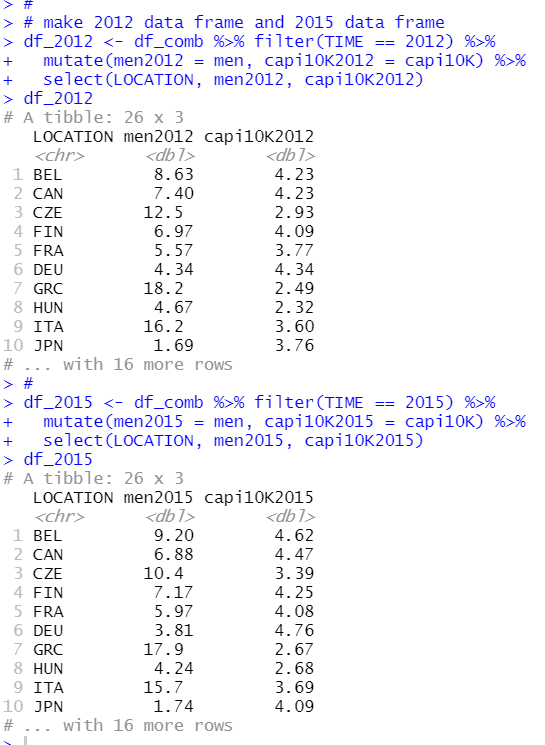Then, I merge the two data frame and make men2015 - men2012 and capi10K2015 - capi10K2012.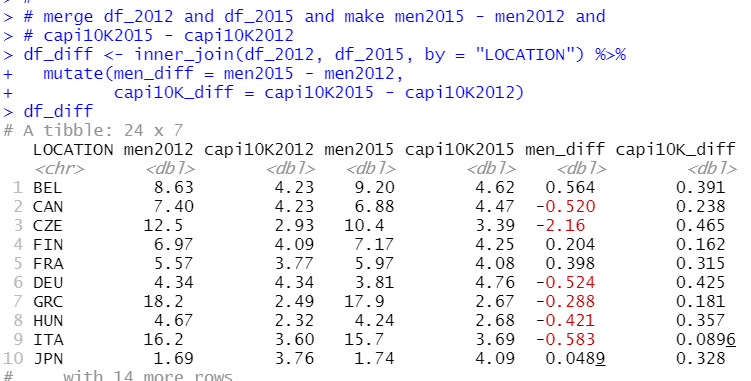All right, let's make regression analysis.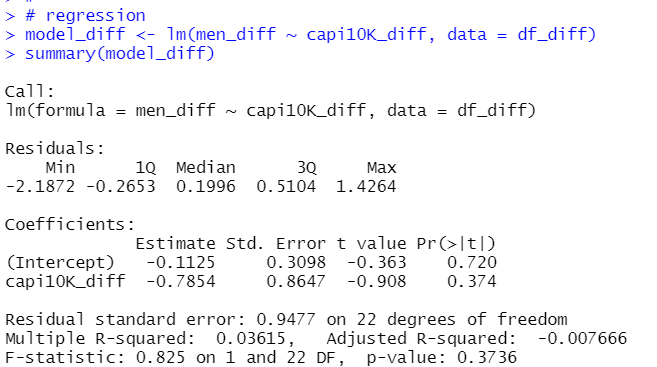coefficient of capi10K_diff is -0.7854, it has smaller impact than previous post's model_1 and model_2. And p-value is greater than 0.05.

So, I cannot say capi10K is significant for men.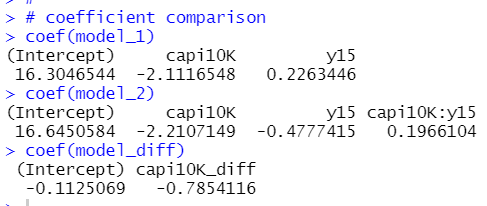Let's draw a sactter plot.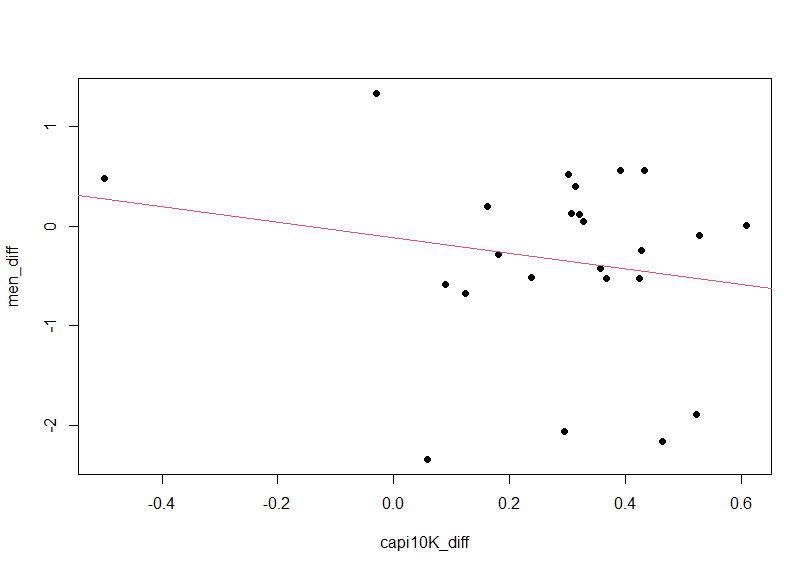Next. I use plm package. It is much convenient for panel data.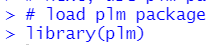Then, I make panel data frame using pdata.frame() function.And I use plm() function with model = "fd" for First Differenced Estimator.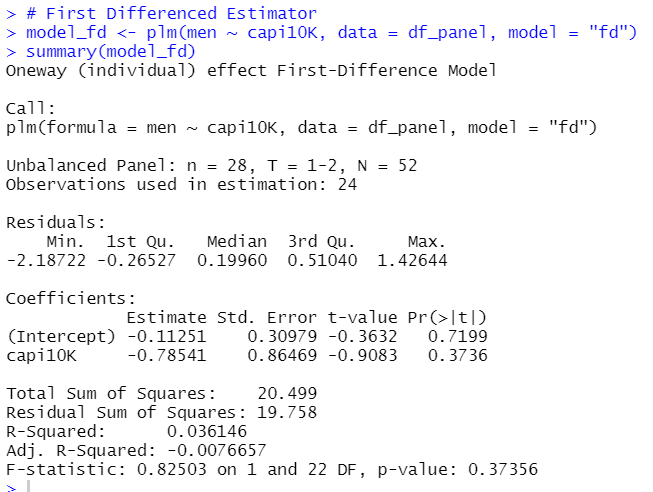capi10K coefficient -0.78541 is the exactly same as model_diff coefficient.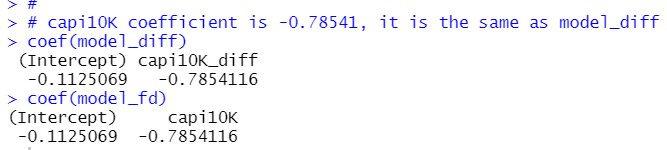That's it. Thank you!